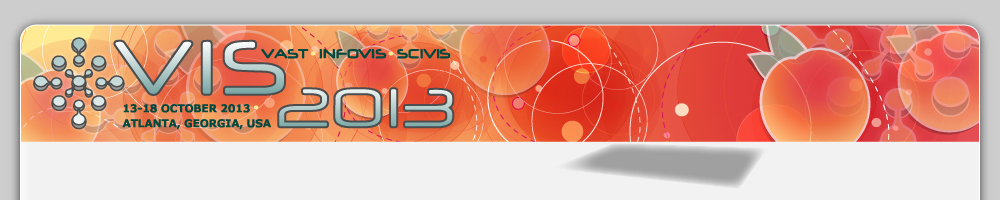13 - 18 OCTOBER 2013, ATLANTA, GEORGIA, USADimension Projection Matrix/Tree: Interactive Subspace Visual Exploration and Analysis of High Dimensional Data

Authors:
Xiaoru Yuan, Donghao Ren, Zuchao Wang, Cong Guo
Abstract:

For high-dimensional data, this work proposes two novel visual exploration methods to gain insights into the data aspect and the dimension aspect of the data. The first is a Dimension Projection Matrix, as an extension of a scatterplot matrix. In the matrix, each row or column represents a group of dimensions, and each cell shows a dimension projection (such as MDS) of the data with the corresponding dimensions. The second is a Dimension Projection Tree, where every node is either a dimension projection plot or a Dimension Projection Matrix. Nodes are connected with links and each child node in the tree covers a subset of the parent nodeﾕs dimensions or a subset of the parent nodeﾕs data items. While the tree nodes visualize the subspaces of dimensions or subsets of the data items under exploration, the matrix nodes enable cross-comparison between different combinations of subspaces. Both Dimension Projection Matrix and Dimension Project Tree can be constructed algorithmically through automation, or manually through user interaction. Our implementation enables interactions such as drilling down to explore different levels of the data, merging or splitting the subspaces to adjust the matrix, and applying brushing to select data clusters. Our method enables simultaneously exploring data correlation and dimension correlation for data with high dimensions.